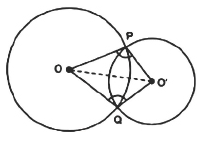Guru

# Prove that the line of centres of two intersecting circles subtends equal angles at the two points of intersection. Q.1

• 0

what is the tricky way for solving the question of class 9th ncert math of Circles chapter of ncert of exercise 10.6of math give me the best and simple way for solving this question in easy and simple way of question no.1 Prove that the line of centres of two intersecting circles subtends equal angles at the two points of intersection

Share

1. Consider the following diagramIn ΔPOO’ and ΔQOO’

OP = OQ          (Radius of circle 1)

O’P = O’Q        (Radius of circle 2)

OO’ = OO’        (Common arm)

So, by SSS congruency, ΔPOO’ ≅ ΔQOO’

Thus, ∠OPO’ = ∠OQO’ (proved).

• 0